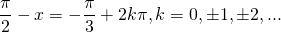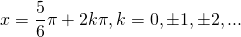# Solution assignment 05 Trigonometric equations

### Assignment 5

Solve the following equatio: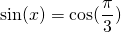### Solution

In the left- and right-hand side there are different functions, a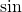- and a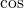-function (actually the latter one is a constant). In order to solve the equation we write: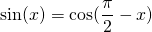getting the equation: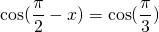and thus we get the following solutions: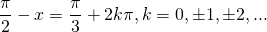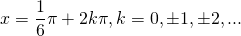or: